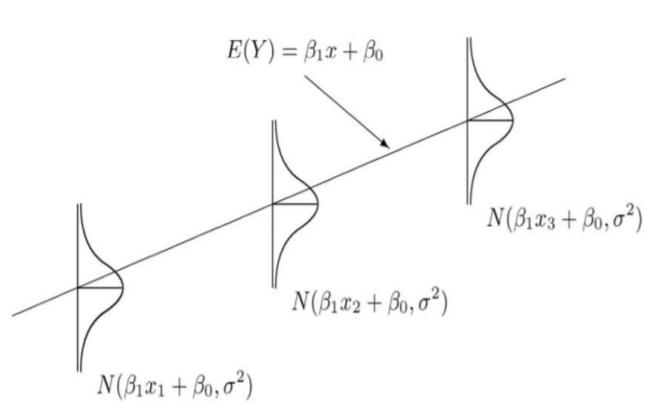# 3.3.5: Estimating $$\sigma$$, the standard error of the residuals

$$\newcommand{\vecs}{\overset { \rightharpoonup} {\mathbf{#1}} }$$ $$\newcommand{\vecd}{\overset{-\!-\!\rightharpoonup}{\vphantom{a}\smash {#1}}}$$$$\newcommand{\id}{\mathrm{id}}$$ $$\newcommand{\Span}{\mathrm{span}}$$ $$\newcommand{\kernel}{\mathrm{null}\,}$$ $$\newcommand{\range}{\mathrm{range}\,}$$ $$\newcommand{\RealPart}{\mathrm{Re}}$$ $$\newcommand{\ImaginaryPart}{\mathrm{Im}}$$ $$\newcommand{\Argument}{\mathrm{Arg}}$$ $$\newcommand{\norm}{\| #1 \|}$$ $$\newcommand{\inner}{\langle #1, #2 \rangle}$$ $$\newcommand{\Span}{\mathrm{span}}$$ $$\newcommand{\id}{\mathrm{id}}$$ $$\newcommand{\Span}{\mathrm{span}}$$ $$\newcommand{\kernel}{\mathrm{null}\,}$$ $$\newcommand{\range}{\mathrm{range}\,}$$ $$\newcommand{\RealPart}{\mathrm{Re}}$$ $$\newcommand{\ImaginaryPart}{\mathrm{Im}}$$ $$\newcommand{\Argument}{\mathrm{Arg}}$$ $$\newcommand{\norm}{\| #1 \|}$$ $$\newcommand{\inner}{\langle #1, #2 \rangle}$$ $$\newcommand{\Span}{\mathrm{span}}$$$$\newcommand{\AA}{\unicode[.8,0]{x212B}}$$The simple linear regression model ($$Y=\beta_{0}+\beta_{1} X+\varepsilon$$) includes a random variable $$\varepsilon$$ representing the residual which follows a Normal Distribution with an expected value of 0 and a standard deviation $$\sigma$$ which is independent of the value of $$X$$. The estimate of  $$\sigma$$ is called the sample standard error of the residuals and is represented by the symbol $$s_e$$. We can use the fact that the Mean Square Error (MSE) from the ANOVA table represents the estimated variance of the residuals errors:

$S_{e}=\sqrt{\mathrm{MSE}}=\sqrt{\dfrac{\mathrm{SSE}}{n-2}} \nonumber$

##### Example: Rainfall and sales of sunglasses

For the rainfall data, the standard error of the residuals is determined as:

$s_{e}=\sqrt{12.859}=3.586 \nonumber$

Keep in mind that this is the standard deviation of the residual errors and should not be confused with the standard deviation of $$Y$$.

3.3.5: Estimating $$\sigma$$, the standard error of the residuals is shared under a CC BY-SA license and was authored, remixed, and/or curated by LibreTexts.